[中文] Search Internet Search HelloDBA
Oracle Technology Site. email: fuyuncat@gmail.com  MSN: fuyuncat@hotmail.com# Full Table Scan cost formula cracking(4)

[中文]

Author:  fuyuncat

Source:  www.HelloDBA.com

Date:  2009-08-01 15:03:34

Share to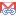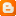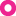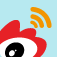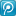### 3.4.11.Max filter column position (with mixed filter column data type)

When I using a mixed filter column data type in the test, I found the effect of MAXFILPOS in the formula is not linear, but changes is still regular:

 date NUMBER MAXFILPOS 5 EFFCOLNUMS 5 FLTCOLNUM resc_cpu CPU_B CPU_A TYPFAC 1 387121440 7121440 380000000 380 2 402121440 7121440 395000000 395 3 402871440 7121440 395750000 395.75 4 402908940 7121440 395787500 395.7875 5 402910815 7121440 395789375 395.78938 date NUMBER MAXFILPOS 4 EFFCOLNUMS 5 FLTCOLNUM resc_cpu CPU_B CPU_A TYPFAC 1 368121440 7121440 361000000 361 19 2 382171440 7121440 375050000 375.05 19.95 3 382873940 7121440 375752500 375.7525 19.9975 4 382909060 7121440 375787620 375.78762 19.99988 date NUMBER MAXFILPOS 3 EFFCOLNUMS 5 FLTCOLNUM resc_cpu CPU_B CPU_A TYPFAC 1 349121440 7121440 342000000 342 19 2 362221440 7121440 355100000 355.1 19.95 3 362876440 7121440 355755000 355.755 19.9975

Observe the data, I finally get the rule:

F(MAXFILPOS)–F(MAXFILPOS-1) = 20–20^(1-FLTCOLNUM)

Put this equation into formula 12, we get the new formula:

Formula 13: TYPFAC = 130 + EFFCOLNUMS + 5*19 - (5-MAXFLTCOLPOS)*(20–20^(1-FLTCOLNUM)) + COLTYPEFAC1*(1/20)^0 + COLTYPEFAC2*(1/20)^1 + … + COLTYPEFACn*(1/20)^(FLTCOLNUM-1)

= 225 + EFFCOLNUMS - (5-MAXFLTCOLPOS)*(20–20^(1-FLTCOLNUM)) + COLTYPEFAC1*(1/20)^0 + COLTYPEFAC2*(1/20)^1 + … + COLTYPEFACn*(1/20)^(FLTCOLNUM-1)

= 225 + EFFCOLNUMS -100 + MAXFLTCOLPOS*20 + (5-MAXFLTCOLPOS)*20^(1-FLTCOLNUM) + COLTYPEFAC1*(1/20)^0 + COLTYPEFAC2*(1/20)^1 + … + COLTYPEFACn*(1/20)^(FLTCOLNUM-1)

= 125 + EFFCOLNUMS + MAXFLTCOLPOS*20 + (5-MAXFLTCOLPOS)*20^(1-FLTCOLNUM) + COLTYPEFAC1*(1/20)^0 + COLTYPEFAC2*(1/20)^1 + … + COLTYPEFACn*(1/20)^(FLTCOLNUM-1)

### 3.4.12.Effective column number (with mixed filter column data type)

Besides MAXFLTCOLPOS, I also found other factor violated the formula we ever got in the query with mixed filter column data type, which is EFFCOLNUM:

 date NUMBER MAXFILPOS 3 EFFCOLNUMS 5 FLTCOLNUM resc_cpu CPU_B CPU_A TYPFAC 1 349121440 7121440 342000000 342 2 362221440 7121440 355100000 355.1 3 362876440 7121440 355755000 355.755 date NUMBER MAXFILPOS 3 EFFCOLNUMS 4 FLTCOLNUM resc_cpu CPU_B CPU_A TYPFAC 1 348121440 7121440 341000000 341 1 2 362171440 7121440 355050000 355.05 0.05 3 362873940 7121440 355752500 355.7525 0.0025 date NUMBER MAXFILPOS 3 EFFCOLNUMS 3 FLTCOLNUM resc_cpu CPU_B CPU_A TYPFAC 1 347121440 7121440 340000000 340 1 2 362121440 7121440 355000000 355 0.05 3 362871440 7121440 355750000 355.75 0.0025

Fortunately, the rule is not hard to be found:

F(EFFCOLNUMS)–F(EFFCOLNUMS-1) = 20^(1-FLTCOLNUM)

Modify formula 13 base on this rule:

Formula 14: TYPFAC = 130+(20*EFFCOLNUM-100)*(20^(-FILTCLNUM))+MAXFLTCOLPOS*20+(5-MAXFLTCOLPOS)*20^(1-FLTCOLNUM)+ COLTYPEFAC1*(1/20)^0 + COLTYPEFAC2*(1/20)^1 + … + COLTYPEFACn*(1/20)^(FLTCOLNUM-1)

= 130 + EFFCOLNUM*20^(1-FILTCOLNUM) - 5*20^(1-FILTCOLNUM) + MAXFLTCOLPOS*20 + (5-MAXFLTCOLPOS)*20^(1-FLTCOLNUM) + COLTYPEFAC1*(1/20)^0 + COLTYPEFAC2*(1/20)^1 + … + COLTYPEFACn*(1/20)^(FLTCOLNUM-1)

= 130 + EFFCOLNUM*20^(1-FILTCOLNUM) + MAXFLTCOLPOS*(20 - 20^(1-FLTCOLNUM))) + COLTYPEFAC1*(1/20)^0 + COLTYPEFAC2*(1/20)^1 + … + COLTYPEFACn*(1/20)^(FLTCOLNUM-1)

= 130 + EFFCOLNUM*20^(1-FILTCOLNUM) + MAXFLTCOLPOS*(20 - 20^(1-FLTCOLNUM))) + COLTYPEFAC1*(1/20)^0 + COLTYPEFAC2*(1/20)^1 + … + COLTYPEFACn*(1/20)^(FLTCOLNUM-1)

= 130 + EFFCOLNUM*20^(1-FILTCOLNUM) + MAXFLTCOLPOS*(20 - 20^(1-FLTCOLNUM))) + COLTYPEFAC1*(1/20)^0 + COLTYPEFAC2*(1/20)^1 + … + COLTYPEFACn*(1/20)^(FLTCOLNUM-1)

Consider formula 8-1, it can be transformed as:

Formula 15: TYPFAC = 130 + (EFFQRYCOLNUM + MAXFLTCOLPOS)*20^(1-FILTCOLNUM) + MAXFLTCOLPOS*(20 - 20^(1-FLTCOLNUM))) + COLTYPEFAC1*(1/20)^0 + COLTYPEFAC2*(1/20)^1 + … + COLTYPEFACn*(1/20)^(FLTCOLNUM-1)

= 130 + EFFQRYCOLNUM*(1/20)^(FILTCOLNUM-1) + MAXFLTCOLPOS*20+ COLTYPEFAC1*(1/20)^0 + COLTYPEFAC2*(1/20)^1 + … + COLTYPEFACn*(1/20)^(FLTCOLNUM-1)

### 3.4.13.Filter columns computing sequence (with mixed filter column data type)

We mentioned before, if the data types of 2 columns be changed in turn, it is difficult to see the rule similar to previous data. So I guess the optimizer will compute the filter columns in a special sequence (it’s the truth, can be controlled by hints, which is out of range of our article), to get the value of the power (the n in COLTYPEFAC2 * (1 / 20) ^ n) above.
A closer observation on the test data with mixed data types, we can still find many other rules (two columns in this example):

 COL1 COL2 MAXFLTPOS COST_CPU varchar2 date 3 262221440 number char 4 274671440 date varchar2 5 302121440 char varchar2 1 269671440 varchar2 number 2 294621440 … … … …

Then put the above data into the formula 12, we found such a rule: when mix CHAR/VARCHAR2 with NUMBER/DATE, the CHAR/VARCHAR2 column has the smaller power value; when fix NUMBER with DATE, NUMBER column will have the smaller power value. Considering their COLTYPEFAC, we can draw such a assumption 9: Optimizer will calculate the AND filter columns in their COLTYPEFAC sequence, from small to big, increasing power value start from 1.

I adopted a more complex combination of mixed data type columns to prove this assumption:

 COLA COLB COLC COLD COLE MAXFLTPOS COST_CPU VARCAHR2 number date varchar2 date 5 290035815 Number varchar2 date date varchar2 5 290035815

In these 2 copies of data, in spite of the physical location of the column, they have the same set of data types: (VARCHAR2, NUMBER, DATE, VARCHAR2, DATE). According to assumption 9, their uniform sequence is (DATE, DATE, NUMBER, VARCHAR2, VARCHAR2), therefore calculated the same TYPEFAC:
130 +20 * 5 +50 * (1 / 20) ^ 0 +50 * (1 / 20) ^ 1 +150 * (1 / 20) ^ 2 +300 * (1 / 20) ^ 3 +300 * (1 / 20) ^ 4 = 282.9144 = 282.914375
The results is same the results calculate from formula 5:
(290035815 - (4500/8192 +0.32) * 1000 * 81929) / 1000000 = 282.914375
Through other random data, we can find that the assumption 9 is valid.

Note: In fact, this sequence rule is not the finally rule, it’s the one we can find under current circumstance. We will find the real rule when I involved more complex conditions.

### 3.4.14.Filter operator (=)

Prior to that, our filter operator is > or <. How about to change it to other operator? We first try on =.

#### 3.4.14.1.Distinct value number of the column

Use the same method and process as > operator, it comes to the formula (here, I did not take query column whose position is greater than the max filter column position column into account, because it’s relatively complexity, I will analysis it in further later):
130 + MAXFLTCOLPOS * 20 + COLTYPEFAC1 * (1 / 1600) ^ 0 + COLTYPEFAC2 * (1 / 1600) ^ 1 + ... + COLTYPEFACn * (1 / 1600) ^ (FLTCOLNUM-1)
Compare to the formula of >, the obvious difference is filter expression in the sub-base, form 1/20 to 1/1600. By reference to the data of baseline table, the result is easily let me associate another test I eve did: column selectivity (see the article ). In that test, there is a preliminary conclusion: when use bind variables, the selectivity of >, <, <= and >=  is 1/20, and the selectivity of = is 1/NDV. Here 1600 is the uniform number of distinct values (NDV) of all of the columns.
By modifying the NDV, it proves that this assumption is valid. Formula can therefore be changed as:

Formula 16: 130 + MAXFLTCOLPOS*20+ COLTYPEFAC1*(1/NDV)^0 + COLTYPEFAC2*(1/NDV)^1 + … + COLTYPEFACn*(1/NDV)^(FLTCOLNUM-1)

#### 3.4.14.2.Mixed NDV

Because the all of the columns of the base table have been set to the same NDV, after come out the formula 16, I naturally think of another question: If the filters have the different NDV, is sub-expression also different? Still look at data with various columns NDV:

 A:160 B:80 C:100 D:200 E:400 FILTER CPU_B CPU_A TYPFAC CACULATED a = :v1 b = :v2 478996440 7121440 471.875 471.875 c = :v3 b = :v2 500121440 7121440 493 493 a = :v1 b = :v2 c = :v3 499015190 7121440 491.89375 491.89375 b = :v2 d = :v4 e = :v5 537875190 7121440 530.75375 530.75375 a = v1 b = :v2 c = :v4 e = :v5 537876174 7121440 530.75473 530.7547344 c = v3 b = :v2 d = :v4 e = :v5 537875228 7121440 530.75379 530.7537875 a = :v1 c = v3 b = :v2 d = :v4 e = :v5 537875214 7121440 530.75377 530.7537737 … …

From these data, the rule can be found: the effective NDV on each filter column is product of NDV of the columns prior to it. For example, under the assumption 9, the fifth data above: the effective NDV of the first column of is 1 (none previous column), the effective NDV of the second column is 400, the effective NDV of the third column is 400 * 200 ... ..., and its computing expression as follows:

30 + 5 * 20 + 300 * (1 / 1) + 300 * (1 / 400) + 300 * (1 / 400) * (1 / 200) + 300 * (1 / 400) * (1 / 200) * (1 / 160) + 300 * (1 / 400) * (1 / 200) * (1 / 160) * (1 / 100) = 530.7537875

According to this rule, the formula changes as follows:

Formula 16: 130 + MAXFLTCOLPOS*20+ COLTYPEFAC1*(1/1) + COLTYPEFAC2*(1/MAXNDV1) + … + COLTYPEFACn*(1/MAXNDV1)*…*(1/MAXNDVn-1)

Note: the formula can also be applied to <, >, while its NDV is 20.

#### 3.4.14.3.Mixed data types

In the derivation before, we have confirmed columns have different data types, it will calculate the filter column by the sequence based on data type factors, from small to big. However, if there are the mixed data types with mixed NDV, what will be the result? Modify the data type of the base table above; get 2 sets of test data:

 A:VARCHAR2 B:DATE C:NUMBER D:NUMBER E:VARCHAR2 FILTER CPU_B CPU_A TYPFAC CACULATED a = :v1 b = :v2 228996440 7121440 221.875 221.875 c = :v3 b = :v2 350121440 7121440 343 343 a = :v1 b = :v2 c = :v3 248077690 7121440 240.95625 240.95625 b = :v2 d = :v4 e = :v5 287500190 7121440 280.37875 280.37875 a = v1 b = :v2 c = :v4 e = :v5 287248831 7121440 280.12739 280.1273906 c = v3 b = :v2 d = :v4 e = :v5 287498353 7121440 280.37691 280.3769125 a = :v1 c = v3 b = :v2 d = :v4 e = :v5 287248796 7121440 280.12736 280.1273557 A:VARCHAR2 B:NUMBER C:DATE D:DATE E:NUMBER FILTER CPU_B CPU_A TYPFAC CACULATED a = :v1 b = :v2 228058940 7121440 220.9375 220.9375 c = :v3 b = :v2 350871440 7121440 343.75 343.75 a = :v1 b = :v2 c = :v3 248082378 7121440 240.96094 240.9609375 b = :v2 d = :v4 e = :v5 387505815 7121440 380.38438 380.384375 a = v1 b = :v2 c = :v4 e = :v5 288061342 7121440 280.9399 280.9399023 c = v3 b = :v2 d = :v4 e = :v5 387505862 7121440 380.38442 380.3844219 a = :v1 c = v3 b = :v2 d = :v4 e = :v5 288061343 7121440 280.9399 280.9399026

From these data, a rule can be get: the compute sequence is first considering COLTYPEFAC, from small to large, and then in accordance with selectivity (1/NDV), from small to large, the effective NDV on each filter column is product of NDV of the columns prior to it. For example, the fifth of the second set data above, the expression is:

130+5*20+150*1+150*(1/400)+300*(1/400)*(1/80)+300*(1/400)*(1/80)*(1/200) = 280.9399026

#### 3.4.14.4.Effective query column

We have said that the column whose location position larger than the max filter column position is effective query column. Operated by =, effective query column will be more complex (in fact, it’s also the case in the < formula, but can not easily be found). Look back to the effective column in the < query formula first:

TYPFAC = 130 + MAXFLTCOLPOS*20+ COLTYPEFAC1*(1/20)^0 + COLTYPEFAC2*(1/20)^1 + … + COLTYPEFACn*(1/20)^(FLTCOLNUM-1) + 20*EFFQRYCOLNUM*(1/20)^FILTCOLNUM

By comparing the = formula, we can image the (1/20) in the expression 20*EFFQRYCOLNUM*(1/20)^FILTCOLNUM is the selectivity of the effective query columns. The testing data prove it’s right:

 FLTCOLUMNS 2 MAXFLTCOLPOS 3 Density: 1/40 EFFQRYCOLNUM COST_CPU CPU_B CPU_A TYPFAC CACULATED 1 504633940 7121440 497512500 497.5125 0.0125 2 504646440 7121440 497525000 497.525 0.025 3 504658940 7121440 497537500 497.5375 0.0375 4 504671440 7121440 497550000 497.55 0.05

However, we find some special case soon,

 MAXFLTPOS: 3 Density 1/200 FLTCOLUMNS 3 EFFQRYCOLNUM COST_CPU CPU_B CPU_A TYPFAC CACULATED 1 498628960 7121440 491507520 491.50752 2E-05 2 498628980 7121440 491507540 491.50754 2E-05 3 498629000 7121440 491507560 491.50756 2E-05 4 498629020 7121440 491507580 491.50758 2E-05 MAXFLTPOS 3 Density 1/800 FLTCOLUMNS 2 EFFQRYCOLNUM COST_CPU CPU_B CPU_A TYPFAC 1 497496480 7121440 490375040 490.37504 4E-05 2 497496520 7121440 490375080 490.37508 4E-05

Studied these cases, I found another rule: the increasing will stop at a threshold. How can we get this threshold? Take it easy, ignore it first, we look at another factor first.

#### 3.4.14.5.Table records number

In the derivation of </> formula, we have come to a conclusion: NDV and the records number of the table will not affect COST_CPU. However, in the = formula, NDV has become proved to be one of the factors, then, we should re-inspected the number records of table.

Note: I have ever tested lots of cases to identify the factor, and will just list a few of them as example:

 rows 1000 tabblks 100 Density 1/80 FLTCOLUMNS 1 EFFQRYCOLNUM COST_CPU CPU_B CPU_A TYPFAC CACULATED 1 1202404 712144 490260 490.26 0.26 2 1202664 712144 490520 490.52 0.26 3 1202924 712144 490780 490.78 0.26

Base on these, I finally get the expression of  effective query column in the formula:

20*ROUND((1/NDV)^FLTCOLNUM*TABROWS,0)/TABROWS

After this, we can continue the job on the threshold; it can be drawn as follow,

20*MAX(1,ROUND((1/NDV)^FLTCOLNUM*TABROWS,0))/TABROWS

Formula 16 is transformed as,

Formula 17: TYPFAC = 130 + MAXFLTCOLPOS*20+ COLTYPEFAC1*(1/1) + COLTYPEFAC2*(1/MAXNDV1) + … + COLTYPEFACn*(1/MAXNDV1)*…*(1/MAXNDVn-1) + 20*EFFQRYCOLNUM*MAX(1,ROUND((1/NDV)^FLTCOLNUM*TABROWS,0))/TABROWS

However, the NDV of all columns in these cases are set as same, we should consider their difference now.

 A:160 B:80 C:100 D:200 E:400 A:VARCHAR2 B:NUMBER C:DATE D:DATE E:NUMBER MAXFILPOS 4 EFFCOLNUMS 4 EFFQRYCOLNUM COST_CPU CPU_B TYPFAC CACULATED 1 275409060 7121440 268.28762 0.00012 2 275409180 7121440 268.28774 0.00012 MAXFILPOS 5 EFFCOLNUMS 5 1 295035835 7121440 287.914395 0.00002 2 295035855 7121440 287.914415 0.00002

The rule is not hard to be found: the effective NDV of effective query columns is the product of the NDV of all filter columns. Base on it, the formula become,

Formula 18: TYPFAC = 130 + MAXFLTCOLPOS*20+ COLTYPEFAC1*(1/1) + COLTYPEFAC2*(1/MAXNDV1) + … + COLTYPEFACn*(1/MAXNDV1)*…*(1/MAXNDVn-1) + 20*EFFQRYCOLNUM*MAX(1,ROUND((1/MAXNDV1)*…*(1/MAXNDVn)*TABROWS,0))/TABROWS

Note: this formula can also be applied to </> case. Base table records number I used before is large (1000000), it quire more than 5 filter columns (ROUND ((1 / 20) ^ 5 * 1000000,0) = 0 <1) to get the threshold, so the threshold had not been noted. We can confirm it also exists in </> case by testing.